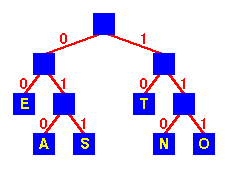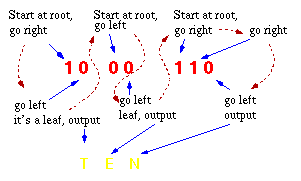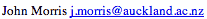Data Structures and Algorithms 11 Huffman Encoding

This problem is that of finding the minimum length bit string which can be used to encode a string of symbols. One application is text compression:

What's the smallest number of bits (hence the minimum size of file) we can use to store an arbitrary piece of text?
Huffman's scheme uses a table of frequency of occurrence for each symbol (or character) in the input. This table may be derived from the input itself or from data which is representative of the input. For instance, the frequency of occurrence of letters in normal English might be derived from processing a large number of text documents and then used for encoding all text documents. We then need to assign a variable-length bit string to each character that unambiguously represents that character. This means that the encoding for each character must have a unique prefix. If the characters to be encoded are arranged in a binary tree:Encoding tree for ETASNO An encoding for each character is found by following the tree from the route to the character in the leaf: the encoding is the string of symbols on each branch followed. For example: ``` String Encoding TEA 10 00 010 SEA 011 00 010 TEN 10 00 110 ```

Notes:

1. As desired, the highest frequency letters - E and T - have two digit encodings, whereas all the others have three digit encodings.
2. Encoding would be done with a lookup table.
A divide-and-conquer approach might have us asking which characters should appear in the left and right subtrees and trying to build the tree from the top down. As with the optimal binary search tree, this will lead to to an exponential time algorithm.

A greedy approach places our n characters in n sub-trees and starts by combining the two least weight nodes into a tree which is assigned the sum of the two leaf node weights as the weight for its root node.

The time complexity of the Huffman algorithm is O(nlogn). Using a heap to store the weight of each tree, each iteration requires O(logn) time to determine the cheapest weight and insert the new weight. There are O(n) iterations, one for each item.

### Decoding Huffman-encoded Data

"How do we decode a Huffman-encoded bit string? With these variable length strings, it's not possible to break up an encoded string of bits into characters!"

 The decoding procedure is deceptively simple. Starting with the first bit in the stream, one then uses successive bits from the stream to determine whether to go left or right in the decoding tree. When we reach a leaf of the tree, we've decoded a character, so we place that character onto the (uncompressed) output stream. The next bit in the input stream is the first bit of the next character.### Transmission and storage of Huffman-encoded Data

If your system is continually dealing with data in which the symbols have similar frequencies of occurence, then both encoders and decoders can use a standard encoding table/decoding tree. However, even text data from various sources will have quite different characteristics. For example, ordinary English text will have generally have 'e' at the root of the tree, with short encodings for 'a' and 't', whereas C programs would generally have ';' at the root, with short encodings for other punctuation marks such as '(' and ')' (depending on the number and length of comments!). If the data has variable frequencies, then, for optimal encoding, we have to generate an encoding tree for each data set and store or transmit the encoding with the data. The extra cost of transmitting the encoding tree means that we will not gain an overall benefit unless the data stream to be encoded is quite long - so that the savings through compression more than compensate for the cost of the transmitting the encoding tree also.

#### Sample Code

A full implementation of the Huffman algorithm is available from Verilib. Currently, there is a Java version there. C and C++ versions will soon be available also.

## Other problems

### Optimal Merge Pattern

We have a set of files of various sizes to be merged. In what order and combinations should we merge them? The solution to this problem is basically the same as the Huffman algorithm - a merge tree is constructed with the largest file at its root.

©, 1998